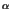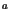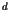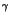Next: 4.5.3.9 Boundary Coefficient Setup Up: 4.5.3 PROMIS-NT Model Classes Previous: 4.5.3.7 Device Setup

### 4.5.3.8 Assembly of Coefficients for Segment Models

A CoefficientSetupModel associates MDL class types with the coefficients of the transport equations (4.1) and (4.2) for the actual segment the model is connected to. Its interface (Table 4.14) consists of associative lists and arrays which assign one MDL class type to the coefficient with the equal name. The indicesandare replaced by the MDL names of the respective quantities as defined in Section 4.5.3.2.

Table 4.14: PROMIS-NT segment coefficient setup Parameters
Name Type Description
alpha XmdModelMatrix
 Associative array specifying thecoefficients
a XmdModelMatrix
 Associative array specifying thecoefficients
b XmdModelMatrix
 Associative array specifying thecoefficients
c XmdModelMatrix
 Associative array specifying thecoefficients
d XmdModelMap
 Associative list specifying thecoefficients
gamma XmdModelMap
 Associative list specifying thecoefficientsNewModel PolyCoeff  : CoefficientSetupModel {
evaluate {
:alpha["As"]["As"] = "Promis_alpha_As_in_Si";
:alpha["B"] ["B"]  = "Promis_alpha_B_in_Si";

:a["As"]["As"]     = "Promis_a_As_in_Si";
:a["B"] ["B"]      = "Promis_a_B_in_Si";

:gamma["As"]       = "Promis_gamma_As_in_Si";
:gamma["B" ]       = "Promis_gamma_B_in_Si";
}
}

Example 4.5: A sample CoefficientSetupModelNext: 4.5.3.9 Boundary Coefficient Setup Up: 4.5.3 PROMIS-NT Model Classes Previous: 4.5.3.7 Device Setup
Robert Mlekus
1999-11-14